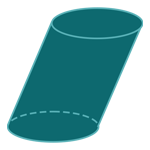Report a Problem
Suggestions

#Calculate The Surface Area of An Oblique Cylinder

Last updated: Saturday, April 29, 2023
Select a cylindrical shape below
Right Cylinder
Hollow Cylinder
Oblique Cylinder
Right Truncated
Cylindrical Wedge

The surface area of an oblique cylinder is the sum of the areas of all its surfaces. An oblique cylinder is a cylinder where the top and bottom circles are not parallel to each other, and its sides are curved rectangles. To find the surface area of an oblique cylinder, you need to calculate the area of each surface separately and then add them together.

Real-life examples of oblique cylinders include soda cans and many types of industrial tanks and storage containers that are tilted or positioned at an angle. The surface area of an oblique cylinder is important to know in industries such as manufacturing and engineering, where these shapes are commonly used in the design and production of products and equipment.

The formula for determining the surface area of an oblique cylinder is defined as:
$$SA$$ $$=$$ $$2$$ $$\cdot$$ $$\pi$$ $$\cdot$$ $$r$$ $$\cdot$$ $$(h_s$$ $$+$$ $$r)$$
$$SA$$: the surface area of the cylinder
$$r$$: the radius of the base
$$h$$: the height of the cylinder
$$\theta$$: the angle of the slope
The SI unit of surface area is: $$square \text{ } meter\text{ }(m^2)$$

## Find $$SA$$

Use this calculator to determine the surface area of an oblique cylinder when the radius of the bases and the height are given.
Hold & Drag
CLOSE
$$r$$
$$meter$$
the height of the cylinder
$$h$$
$$meter$$
the angle of the slope
$$\theta$$
$$degree$$
Bookmark this page or risk going on a digital treasure hunt again